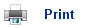# Predicting the direction of Redox Reactions

• Redox reactions involve the transfer of electrons.
• The oxidising agent is reduced (i.e. it gains electrons; it is the “electron thief”).
• The reducing agent is oxidised (i.e. it loses electrons; it is the “electron donator”).
• Redox reactions can be written in two half reactions; one reaction producing the electrons and the other reaction accepting them.
• For example, when Zinc is added to copper sulphate, the following reaction occurs: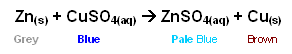• In addition to just the colour change, the reaction is also exothermic and so results in an increase in temperature.
• The reaction can be simplified; the sulphate ion is a spectator ion and so can be removed.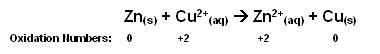• From the oxidation numbers, it is plain to see that the zinc is being oxidised (increase in oxidation number) and the copper is being reduced (decrease in oxidation number).
• The two half equations are:

Cu2+(s) + 2e-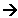Cu(aq) (reduction)
Zn(s)Zn2+(aq) + 2e- (oxidation)

• The reverse reaction of this can not occur; if copper is added to zinc sulphate solution, nothing will happen.
• However, if copper is added to silver nitrate solution, the copper does react: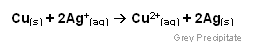• The half equations are:

2Ag+(s) + 2e-2Ag(s) (reduction)
Cu(s)Cu2+(aq) + 2e- (oxidation)

• Once again the reverse reaction cannot occur.
• However; as you can see from the two previous reactions, the individual half reactions are reversible:

Cu(s)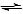Cu2+(aq) + 2e-

• The actual direction that the reaction takes depends upon what it is reacting with. Copper ions are reduced by zinc (the zinc supplies electrons); however copper is oxidised by silver ions (the copper provides electrons).
• Copper atoms don’t transfer electrons to zinc ions, and silver atoms don’t transfer electrons to copper ions.
• Something must control the direction of electron transfer; to find out which way the electrons are transferred requires study of the individual half-equations, which can be done by separating the half-reactions using an electrochemical cell.

## Useful books for revision

Revise A2 Chemistry for Salters (OCR A Level Chemistry B)Salters (OCR) Revise A2 ChemistryHome